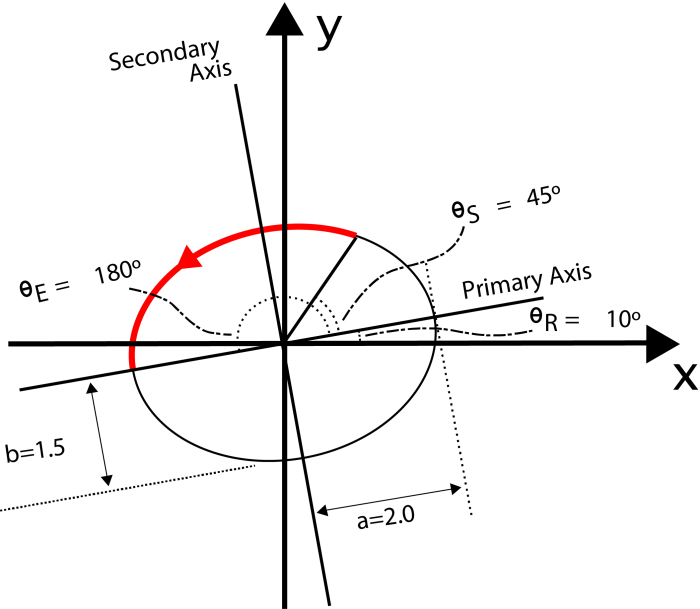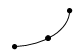# Arcs (IFMEArc)

There are 3 ways to represent an Arc in FME.

## ArcByCenterPoint

Arc features of this type possess an x/y coordinate that represents their center point, plus a mathematical definition of the arc radius and sweep angles. Arcs may be elliptical, in that they may have differing primary and secondary radius values. They may also have a rotation.The Cartesian equation for the ellipse defines the curve by a simple parametric form, with the x and y coordinates having different scalings:

x = a cos(t)

y = b sin(t)

where

Note that `t` is a parameter that does not have a direct interpretation in terms of an angle. However, the relationship between the polar angle (theta) from the ellipse center and the parameter` t` follows from:

theta = arctan((b/a)*tan(t))

or

t = arctan((a/b)*tan(theta))

Example: To specify the red arc in the above diagram in the counterclockwise direction, the following values would need to be set on the feature:

Primary Radius = a = 2.0

Secondary Radius = b = 1.5

Rotation = thetaR = 10 (degrees)

Start Angle = tS = arctan((a/b)*tan(thetaS)) = arctan((2.0/1.5)*tan(45)) = 53.130102354155978703144387440907

Sweep Angle = tE - tS = arctan((a/b)*tan(thetaE)) - tS = arctan((2.0/1.5)*tan(180)) - tS = 180.0 - tS = 180.0 - 53.130102354155978703144387440907 = 126.86989764584402129685561255909

Optionally, the arc may have explicit end points assigned to it.

If the arc is 3D or has measures, the location of the z or measure values is defined as follows:

• If the arc has explicit endpoints, the center point is 2D with no measures and the endpoints may have unique z and/or measure values. The endpoints must both be 2D or both be 3D and must have the same number and named measures, but the values of any of these between them may differ.
• If the arc has no explicit endpoints, the center point may have a single z and/or measure values which are intended to apply to the arc as a whole.

## ArcByBulge

An alternate arc definition is a bulge value, plus the two unique end point coordinates. This definition can only represent circular, unclosed arcs.

The bulge value must be between -1.0 and 1.0, and specifies the curvature of the arc between the two points.If the arc is 3D or has measures, the endpoints may have unique z and/or measure values. The endpoints must both be 2D or both be 3D, and must have the same number and named measures, but the values of any of these between them may differ.

## ArcBy3Points

A further arc definition is the two end points, plus the mid-point of the arc 'line'. (The mid-point does not need to be exactly halfway, but at least somewhere along the arc.) All three points must be unique. This definition can only represent circular, unclosed arcs.If the arc is 3D or has measures, all three points may have unique z and/or measure values. The points must all be 2D or all be 3D, and must have the same number and named measures, but the values of any of these between them may differ.